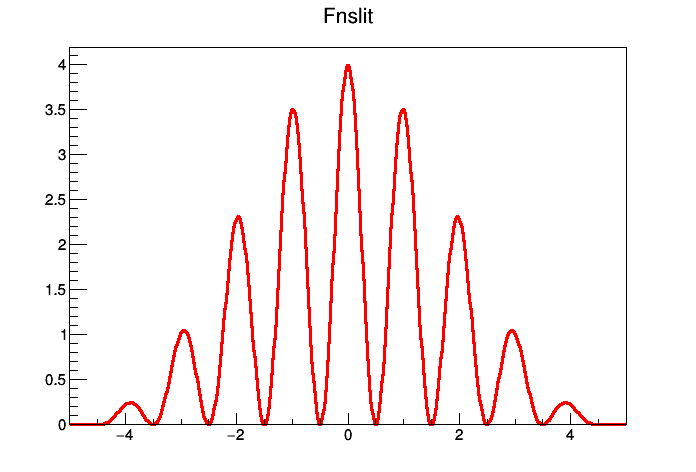# Tutorial: Drawing the interference pattern of light falling on a grid with n slits and a ratio of r of slit width over distance between slits in C++¶

Translation to notebook by: Theis Hansen

In this tutorial, we are going to learn to code the aforementioned grid in C++ To begin with, we need to import ROOT so that we can use the toolkit. We also set our Notebook to C++

In :
from ROOTaaS.iPyROOT import ROOT
ROOT.toCpp()

Welcome to ROOTaas Beta

Notebook is in Cpp mode


### Writing the Function¶

We use ROOT Namespace TMath to access the mathimatical toolkit of ROOT. In this first line we are setting the value for our constant "pi", and we use the math toolkit to state that our "pi" is the mathimatical constant "Pi".

In :
auto pi = TMath::Pi();


Here we are writing the code for our functions that we will call on later. The "*"s are pointers, that access a certain area of the memory (only in the first set of parentheses of each line, the others are multiplications).

The ".dcl" marks a cell as used for declaration of functions.

In :
.dcl
double single(double *x, double *par){return pow(sin(pi*par*x)/(pi*par*x),2);};
double nslit0(double *x, double *par){return pow(sin(pi*par*x)/sin(pi*x),2);};
double nslit(double *x, double *par){return single(x,par) * nslit0(x,par);};


We set the number of slits to 4, and the ration of slit width to distance between slits to 2.

In :
float r=.2,ns=2;


Here we are using the ROOT Type TF1 to write our function and we add the "*" pointer before our Fnslit object so that the information can be saved to the computer's memory. We set the options for our function with the command SetNpx. Here we use the "->" because we are setting a command to an object with a pointer.

In :
TF1 Fnslit("Fnslit",nslit,-5.001,5.,2);
Fnslit.SetNpx(500);


Here you can see that we are setting the parameters for the code, so that we can apply them as fixed variables that we state at the beginning of our code. This saves us the trouble of entering long numbers over and over again. Note that the parameter number starts at 0, not 1.

In :
Fnslit.SetParameters(r,ns);


Now that we've got our code nicely written out, we can use the "Draw" command so that we can see the graph.

In :
Fnslit.Draw();

Info in <TCanvas::MakeDefCanvas>:  created default TCanvas with name c1In [ ]: